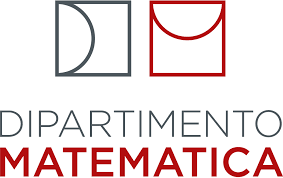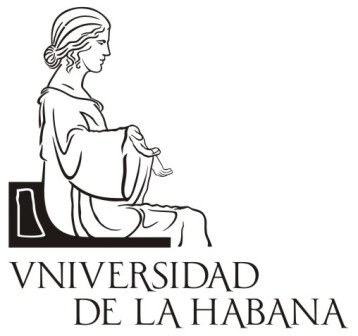# Selected topics in Probability and Statistics @ Habana

## Facultad de Matemática y Computación Universidad de La Habana, Cuba January 28 - February 8, 2019

#### Dates

 Deadline for application: Dec 15, 2018 Notification of acceptance: Dec 23, 2018 School: Jan 28 - Feb 8, 2019

#### Description

The school, at its third edition, is structured into three courses spanned over two weeks, and will deal with some selected themes of Probability and Statistics with a specific attention to the applications in Computer Science and Big Data. Couses are mainly addressed to master students, though open and potentially interesting to PhD students and young researchers. Each course is structured in five lectures, supported by teaching material and by some tutorial lectures, with the aim of introducing students to the basics of some selected research topics, and of providing them the main tools to work in advanced applications.

#### Courses

The lecture program comprises the following three courses:
• [MCA] Markov Chains and selected applications - Alessandra Bianchi
Markov chains and processes are among the most common classes of stochastic processes employed in applications to model the dynamics of complex systems. Their wide use is due, on one hand, to the simplicity of their definition and implementation, and on the other to the accuracy in reproducing the behavior of real systems. The aim of the course is to provide the basics of Markov theory, in order to present and work with some very famous and important application, such as the Monte Carlo methods and the queuing theory. In details, we will first review the definition and the basic properties of Markov chains, from the classification of states to the definition of the stationary distribution and the ergodic theorem. As an application of these results we will present the Monte Carlo methods, that are a class of computational algorithms based on Markov chains and commonly used to sample from a given distribution and in particular in optimization problems. In the second part of the course we will move to continuous time processes with the goal of defining Markov chain in continuous time. We will first introduce the Poisson process, that is a counting process (with huge applications in telecommunications) and that can be easily defined as a point process on R with inter-distances given by i.i.d. exponential random variables. The Poisson process will be the basic tool to move from discrete time to continuous time Markov chains. At last, as an application of Markov chains in continuous time, we will introduce some important model of the queueing theory. Depending on the time and interest, we will descrive the following models: M/M/1, M/M/N, M/M/1/N.
• [Sim] Simulation of random variables and processes - Martino Grasselli
The course deals with simulation, and extremely powerful tool that allows to treat random variables and in general stochastic processes for which few (or even no) analytical methods are available. Methods for generating the values of arbitrarily distributed random variables are discussed. As our starting point in the simulation of random variables from an arbitrary distribution, we shall suppose that we can simulate from the uniform (0,1) distribution, and we shall use the term random numbers to mean independent random variables from this distribution. First we present general techniques for simulating continuous random variables, such as the inverse transformation, the rejection and the hazard rate methods. Some ad-hoc special techniques for simulating the most celebrated random variables, like the exponential, normal, Chi-squared distribution, are also illustrated. Then we proceed to simulate discrete random variables, like e.g. the geometric, binomial and Poisson distributions. There are also rejection and hazard rate methods for discrete distributions, but in the special case of finite discrete random variables there is a simulation technique - called the alias method which, though requiring some setup time, is very fast to implement. Particular attention is given to the simulation of nonhomogeneous Poisson processes, and in fact three different simulation approaches are discussed. We discuss various methods for increasing the precision of the simulation estimates by reducing their variance. Using antithetic variables or control variates, it is possible to reduce sensibly the noise in the simulation. We consider important application in queueing systems. Finally, we describe the importance sampling technique, and we consider the problem of choosing the number of simulation runs needed to attain a desired level of precision. According to the remaining time and the interest of the students, we can consider some financial applications in the problem of option pricing.
• [SBG] Statistical methods for big data - Laura Ventura
The course will focus on the following topics:
• Linear and generalised linear predictive models: Linear Regression Models; Logistic Regression; Inference; Accuracy of a Model; Variable selection.
• Classification methods: Logistic regression methods; Linear discriminant analysis.
• Model selection: R^2, AIC, BIC; Automatic Model Selection Techniques.
• Some extensions: Regression Splines and GAM; Ridge Regression and Lasso.
• Tree-based methods (depending on time and on students interest).

#### Schedule

 1°week (28/01 - 01/02) Monday Tuesday Wednesday Thursday Friday 10-12 MCA MCA MCA MCA MCA 12-13.30 lunch lunch lunch lunch lunch 13.30-15.30 Sim Sim Sim Sim Sim 15.30-16 break break break break break 16-17.30 tutor tutor tutor tutor tutor

 2°week (04/02 - 08/02) Monday Tuesday Wednesday Thursday Friday 10-12 SBD SBD SBD SBD Exams SBD 12-13.30 lunch lunch lunch lunch Closing 13.30-15 tutor tutor SBD tutor -- 15.30-17.30 Exams MCA Exams Sim tutor -- --

#### Organizers

Alessandra Bianchi (Dipartimento di Matematica, Universià di Padova, Italy)
Alberto Fernández Oliva (Facultad de Matemática y Computación, Universidad de La Habana, Cuba)
Vivian R. Sistachs Vega (Facultad de Matemática y Computación, Universidad de La Habana, Cuba)

#### Contacts

For information please mail the organisers.